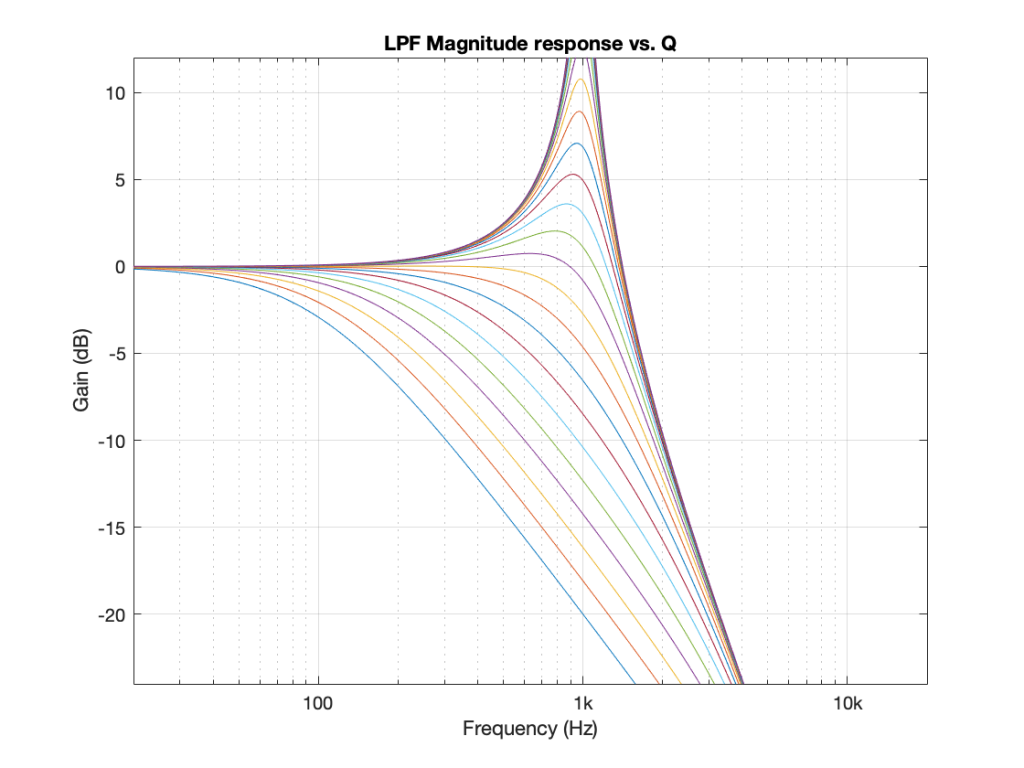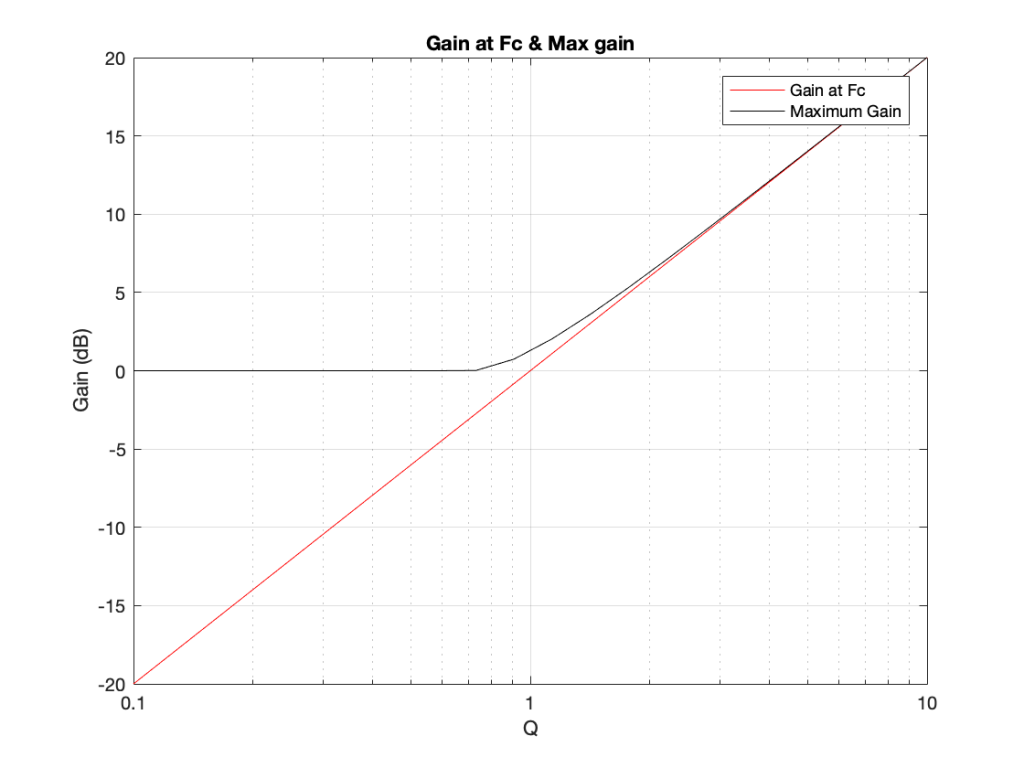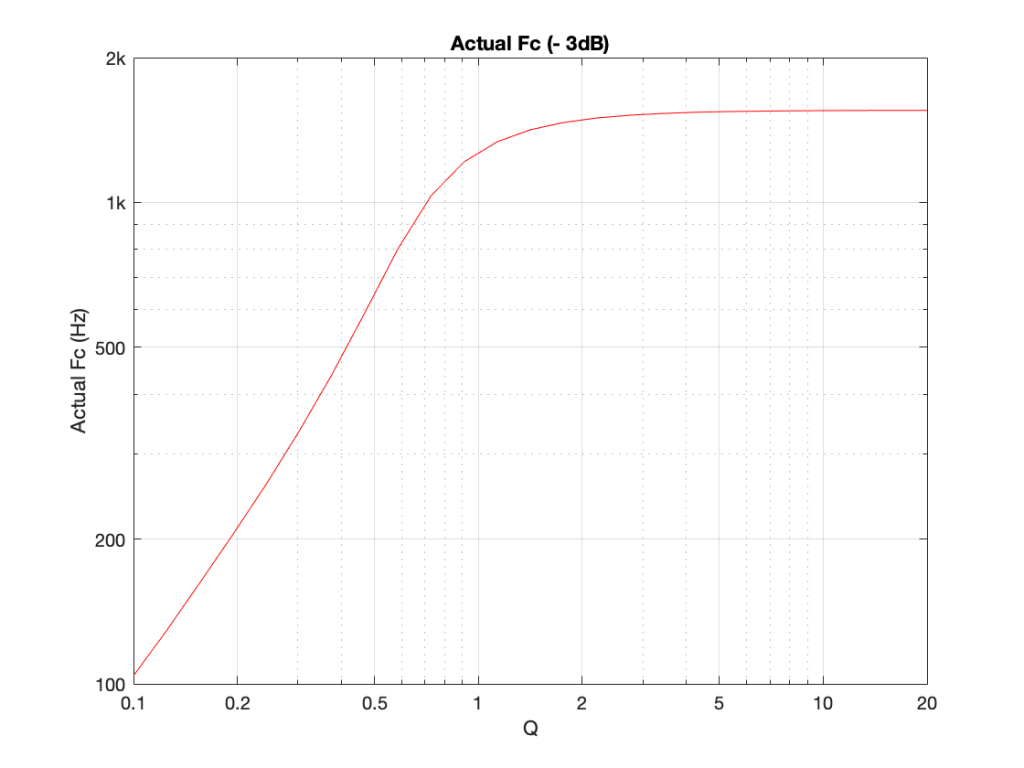# Fc ≠ Fc

I was working on the sound design of a loudspeaker last week with some new people and software – so we had to get some definitions straight before we messed things up by thinking that we were using the same words to mean the same thing. I’ve made a similar mistake to this before, as I’ve written about here – and I don’t being reminded of my own stupidity repeatedly… (Or, as Stephen Wright once said “I’m having amnesia and deja vu at the same time – I think I’ve forgotten this before…”)

So, in this case on that day, we were talking about the lowly 2nd-order Low Pass Filter, based on a single biquad.

If you read about how to find the cutoff frequency of a low-pass filter, you’ll probably find out that you find the frequency where the gain is one half of the power of that in the bandpass portion of the filter’s response. Since 10*log10(0.5) = -3.01 dB, then this is also called the “3 dB down point” of the filter.

In my case, when I’m implementing a filter, I use the math provided by Robert Bristow-Johnson to calculate my biquad coefficients. You input a cutoff frequency (Fc), and a Q value, and (for a given sampling rate) you get your biquad coefficients.

The question then, is: is the desired cutoff frequency the actual measurable cutoff frequency of the system? (Let’s assume for the purposes of this discussion that there are no other components in the system that affect the magnitude response – just to keep it simple.)

The simple answer is: No.

For example, if I make a 2nd-order low pass filter with a desired cutoff frequency of 1 kHz (using a high enough sampling rate to not introduce any errors due to the bilinear transform) and I vary the Q from something very small (in this example, 0.1) to something pretty big (in this example, 20) I get magnitude response curves that look like the figure below.Magnitude responses of 2nd order low pass filters with Q’s ranging from 0.1 to 20.

It is probably already evident that these 25 filter responses plotted above that they do not all cross each other at the 1 kHz line. In addition, you may notice that there is only one of those curves that is -3.01 dB at 1 kHz – when the Q = 1/sqrt(2) or 0.707.

This begs the question: what is the gain of each of those filters at the desired value of Fc (in this case, 1 kHz)? This is plotted as the red line in the figure below.The actual gain value of the filters at the desired Fc, and the maximum gain at any frequency.

This plot also shows the maximum gain of the filters for different values of Q. Notice that, in the low end, the maximum value is 0 dB, since the low pass filters only roll off. However, for Q values higher than 1/sqrt(2), there is an overshoot in the response, resulting in a boost at some frequency. As the Q increases, the frequency at which the gain of the filter is highest approaches the desired cutoff frequency. (As can be seen in the plot above, by the time you get to a Q of 20, the gain at Fc and the maximum gain of the filter are the same.)

It may be intuitively interesting (or interestingly intuitive) to note that, when Q goes to infinity, the gain at Fc also goes to infinity, and (relatively speaking) all other frequencies are infinitely attenuated – so you have a sine wave generator.

So, we know that the gain value at the stated Fc is not -3 dB for all but one value of Q. So, what is the -3 dB point, if we state a desired Fc of 1 kHz and we vary the Q? This is shown in the figure below.The -3 dB point of a 2nd order 1 kHz low pass filter as a function of Q.

So, varying the Q from 0.1 to 20 varies the actual Fc (or, at least, the -3 dB point) from about 104 Hz to about 1554 Hz.

Or, if we plot the same information as a function (or just a multiple) of the desired Fc, you get the plot below.

So, if you’re sitting in a meeting, and the person in front of you is looking at a measurement of a loudspeaker magnitude response, and they say “could you please put in a low pass filter with a cutoff frequency of 1 kHz and a Q of 0.5” you should start asking questions by what, exactly, they mean by “cutoff frequency”… If not, you might just wind up with nice-looking numbers but strangely-sounding loudspeakers.